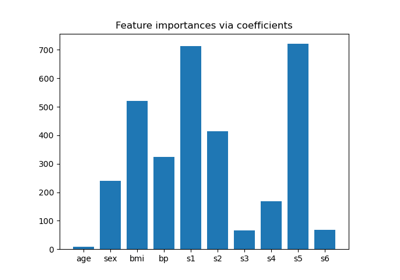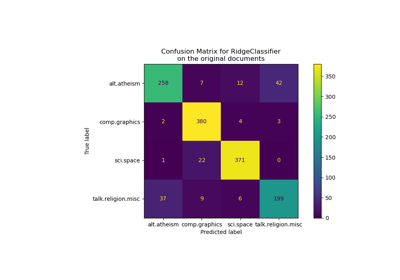# sklearn.feature_selection.SelectFromModel¶

class sklearn.feature_selection.SelectFromModel(estimator, *, threshold=None, prefit=False, norm_order=1, max_features=None, importance_getter='auto')[source]

Meta-transformer for selecting features based on importance weights.

New in version 0.17.

Read more in the User Guide.

Parameters
estimatorobject

The base estimator from which the transformer is built. This can be both a fitted (if prefit is set to True) or a non-fitted estimator. The estimator must have either a feature_importances_ or coef_ attribute after fitting.

thresholdstring or float, default=None

The threshold value to use for feature selection. Features whose importance is greater or equal are kept while the others are discarded. If “median” (resp. “mean”), then the threshold value is the median (resp. the mean) of the feature importances. A scaling factor (e.g., “1.25*mean”) may also be used. If None and if the estimator has a parameter penalty set to l1, either explicitly or implicitly (e.g, Lasso), the threshold used is 1e-5. Otherwise, “mean” is used by default.

prefitbool, default=False

Whether a prefit model is expected to be passed into the constructor directly or not. If True, transform must be called directly and SelectFromModel cannot be used with cross_val_score, GridSearchCV and similar utilities that clone the estimator. Otherwise train the model using fit and then transform to do feature selection.

norm_ordernon-zero int, inf, -inf, default=1

Order of the norm used to filter the vectors of coefficients below threshold in the case where the coef_ attribute of the estimator is of dimension 2.

max_featuresint, default=None

The maximum number of features to select. To only select based on max_features, set threshold=-np.inf.

New in version 0.20.

importance_getterstr or callable, default=’auto’

If ‘auto’, uses the feature importance either through a coef_ attribute or feature_importances_ attribute of estimator.

Also accepts a string that specifies an attribute name/path for extracting feature importance (implemented with attrgetter). For example, give regressor_.coef_ in case of TransformedTargetRegressor or named_steps.clf.feature_importances_ in case of Pipeline with its last step named clf.

If callable, overrides the default feature importance getter. The callable is passed with the fitted estimator and it should return importance for each feature.

New in version 0.24.

Attributes
estimator_an estimator

The base estimator from which the transformer is built. This is stored only when a non-fitted estimator is passed to the SelectFromModel, i.e when prefit is False.

threshold_float

The threshold value used for feature selection.

RFE

Recursive feature elimination based on importance weights.

RFECV

Recursive feature elimination with built-in cross-validated selection of the best number of features.

SequentialFeatureSelector

Sequential cross-validation based feature selection. Does not rely on importance weights.

Notes

Allows NaN/Inf in the input if the underlying estimator does as well.

Examples

>>> from sklearn.feature_selection import SelectFromModel
>>> from sklearn.linear_model import LogisticRegression
>>> X = [[ 0.87, -1.34,  0.31 ],
...      [-2.79, -0.02, -0.85 ],
...      [-1.34, -0.48, -2.55 ],
...      [ 1.92,  1.48,  0.65 ]]
>>> y = [0, 1, 0, 1]
>>> selector = SelectFromModel(estimator=LogisticRegression()).fit(X, y)
>>> selector.estimator_.coef_
array([[-0.3252302 ,  0.83462377,  0.49750423]])
>>> selector.threshold_
0.55245...
>>> selector.get_support()
array([False,  True, False])
>>> selector.transform(X)
array([[-1.34],
[-0.02],
[-0.48],
[ 1.48]])


Methods

 fit(X[, y]) Fit the SelectFromModel meta-transformer. fit_transform(X[, y]) Fit to data, then transform it. get_params([deep]) Get parameters for this estimator. get_support([indices]) Get a mask, or integer index, of the features selected Reverse the transformation operation partial_fit(X[, y]) Fit the SelectFromModel meta-transformer only once. set_params(**params) Set the parameters of this estimator. Reduce X to the selected features.
fit(X, y=None, **fit_params)[source]

Fit the SelectFromModel meta-transformer.

Parameters
Xarray-like of shape (n_samples, n_features)

The training input samples.

yarray-like of shape (n_samples,), default=None

The target values (integers that correspond to classes in classification, real numbers in regression).

**fit_paramsOther estimator specific parameters
Returns
selfobject
fit_transform(X, y=None, **fit_params)[source]

Fit to data, then transform it.

Fits transformer to X and y with optional parameters fit_params and returns a transformed version of X.

Parameters
Xarray-like of shape (n_samples, n_features)

Input samples.

yarray-like of shape (n_samples,) or (n_samples, n_outputs), default=None

Target values (None for unsupervised transformations).

**fit_paramsdict

Returns
X_newndarray array of shape (n_samples, n_features_new)

Transformed array.

get_params(deep=True)[source]

Get parameters for this estimator.

Parameters
deepbool, default=True

If True, will return the parameters for this estimator and contained subobjects that are estimators.

Returns
paramsdict

Parameter names mapped to their values.

get_support(indices=False)[source]

Get a mask, or integer index, of the features selected

Parameters
indicesbool, default=False

If True, the return value will be an array of integers, rather than a boolean mask.

Returns
supportarray

An index that selects the retained features from a feature vector. If indices is False, this is a boolean array of shape [# input features], in which an element is True iff its corresponding feature is selected for retention. If indices is True, this is an integer array of shape [# output features] whose values are indices into the input feature vector.

inverse_transform(X)[source]

Reverse the transformation operation

Parameters
Xarray of shape [n_samples, n_selected_features]

The input samples.

Returns
X_rarray of shape [n_samples, n_original_features]

X with columns of zeros inserted where features would have been removed by transform.

partial_fit(X, y=None, **fit_params)[source]

Fit the SelectFromModel meta-transformer only once.

Parameters
Xarray-like of shape (n_samples, n_features)

The training input samples.

yarray-like of shape (n_samples,), default=None

The target values (integers that correspond to classes in classification, real numbers in regression).

**fit_paramsOther estimator specific parameters
Returns
selfobject
set_params(**params)[source]

Set the parameters of this estimator.

The method works on simple estimators as well as on nested objects (such as Pipeline). The latter have parameters of the form <component>__<parameter> so that it’s possible to update each component of a nested object.

Parameters
**paramsdict

Estimator parameters.

Returns
selfestimator instance

Estimator instance.

transform(X)[source]

Reduce X to the selected features.

Parameters
Xarray of shape [n_samples, n_features]

The input samples.

Returns
X_rarray of shape [n_samples, n_selected_features]

The input samples with only the selected features.

## Examples using sklearn.feature_selection.SelectFromModel¶